# EOMONTH function – generate a sequence of dates

You will sometimes need to generate a sequence of dates in Excel. Most often, you will need month-end dates, but no matter what kind of dates you need, the simplest way to generate them will include the EOMONTH function.

The EOMONTH function syntax is as follows:

= EOMONTH ( start_date; number of months )

Start date is any given date, such as 31.12.2020.

Number of months is an integer number of whole months before or after start_date.

If that number is zero, the end of the start_date month date will be returned.

If that number is 1, the end of the month following the start_date month will be returned.

If that number is -1, the end of the month preceding the start_date month will be returned.

Given that, generating a series of end-of month dates is easy: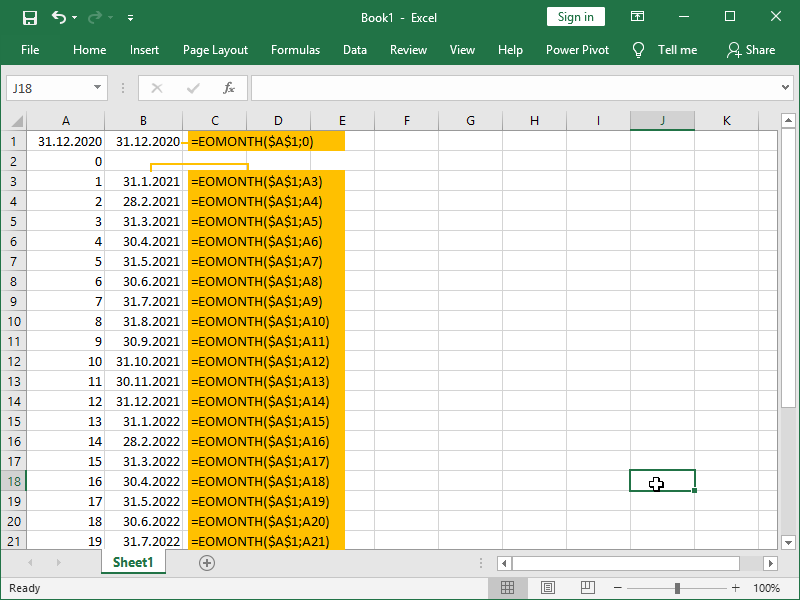If we want to generate a sequence of the start of month dates, we can do that by adding one more day to the month-end date of the previous month: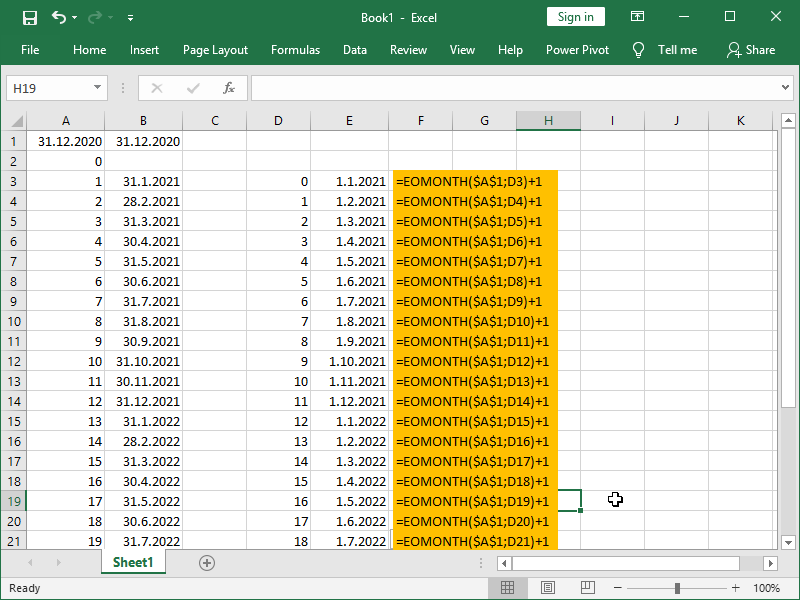If we want to generate a sequence of dates containing every 10th day of the month, we can do that by adding 10 more days to the month-end date of the previous month: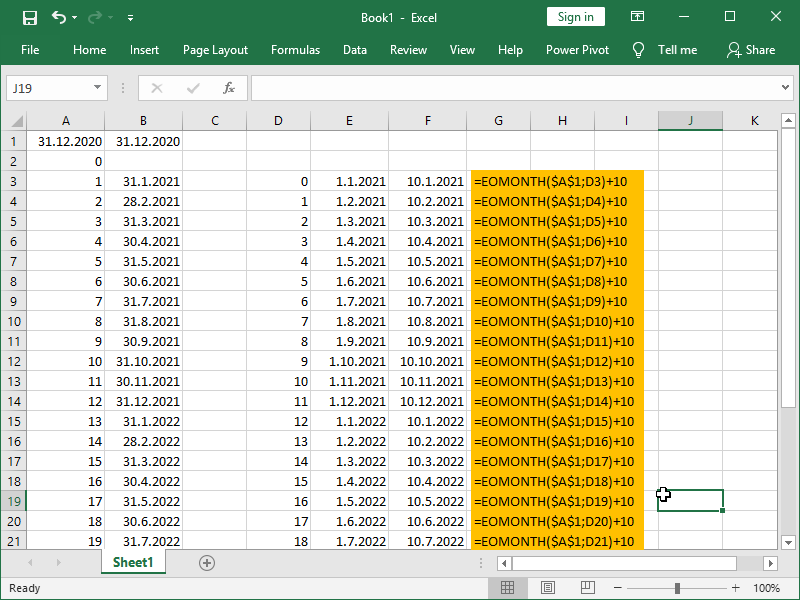If we want to generate a sequence of dates containing every 5th day from the end of the month, we can do that by removing 4 days from the month-end date: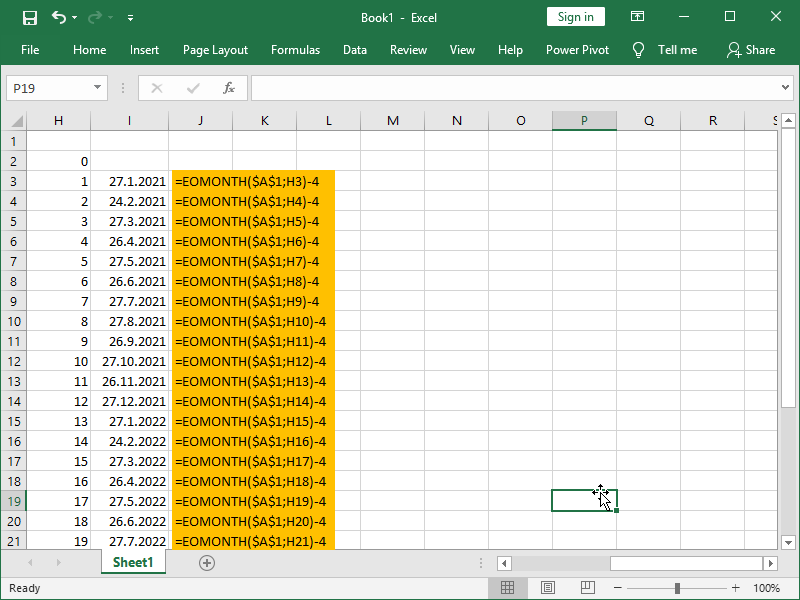If we want to generate a sequence of dates containing every 10th of January, we can do that by manipulating the number of months and adding 10 more days to the month-end date of the previous month: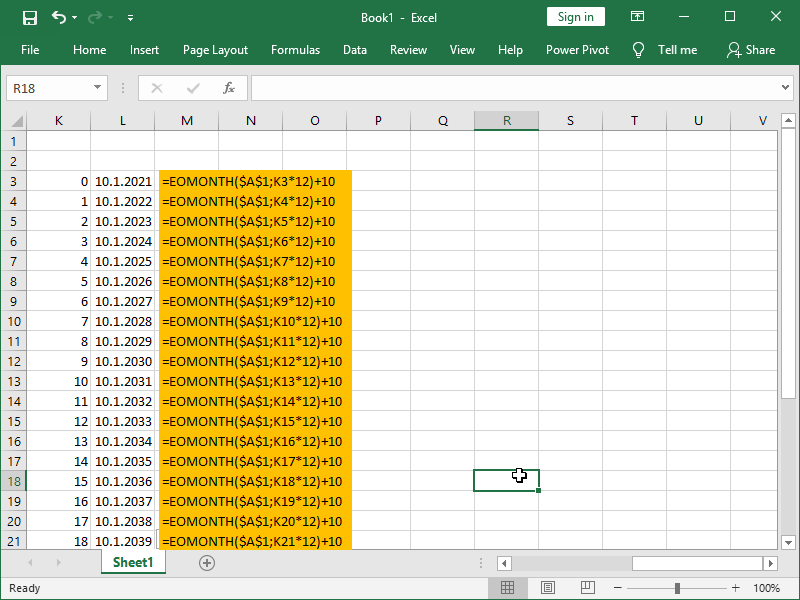Dig deeper:

EOMONTH function – calculate past or future date from the starting date

Date and Time in Excel